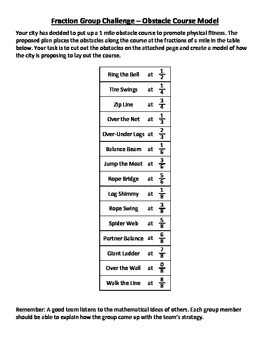# Fractions on a Number Line - Obstacle Course TaskSubject
Resource Type
File Type

PDF

(527 KB|4 pages)
Standards
• Product Description
• StandardsNEW
This task requires students to use their knowledge of fractions to place obstacles on the correct location on an obstacle course. Students must partition the number line using a ruler to help them. Students will realize that some fractions may be on the same point on a number line. This task requires students bring in their knowledge of measurement, multiplication, and division as well as fractions. (Note: Use calculator tape or other 6 foot long material as the obstacle course / number line.)

Aligned with these standards:

3.NF.2
Represent a fraction 1/b on a number line diagram by defining the interval from 0 to 1 as the whole and partitioning it into b equal parts. Recognize that each part has size 1/b and that the endpoint of the part based at 0 locates the number 1/b on the number line.

Represent a fraction a/b on a number line diagram by marking off a lengths 1/b from 0. Recognize that the resulting interval has size a/b and that its endpoint locates the number a/b on the number line.

3.NF.3
Understand two fractions as equivalent (equal) if they are the same size, or the same point on a number line.
Explain equivalence of fractions in special cases, and compare fractions by reasoning about their size.
Understand a fraction as a number on the number line; represent fractions on a number line diagram.
Total Pages
4 pages
N/A
Teaching Duration
1 hour
Report this Resource to TpT
Reported resources will be reviewed by our team. Report this resource to let us know if this resource violates TpT’s content guidelines.